# Boolean algebra with operators

(diff) ← Older revision | Latest revision (diff) | Newer revision → (diff)

An operator on a Boolean algebra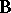is a finitary operation on the Boolean algebra that is additive, meaning that in each of its arguments it preserves the sum/join operation of. An operator is normal if each argument preserves the least element of. Major examples of normal operators are as follows.

Relation algebras: the algebra of binary relations on a set(i.e. the set of subsets of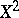) has the binary operator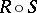of composition of relations and the unary inverse operator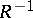. The axiomatic study of these operators quickly leads to deep questions about representability of equationally defined abstract algebras as concrete algebras of relations (cf. [a8], [a9]).

Cylindric algebras: on the algebra of subsets of a Cartesian product set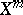, let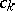be the operator assigning to each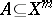the cylinder generated by translatingparallel to the-th coordinate axis. Ifis defined by a formula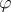having variables, then the cylinderis defined by the existentially quantified formula. This observation leads to a comprehensive algebraic theory with many applications to quantificational logic [a6]. A related theory of polyadic algebras, due to P.R. Halmos [a5], emphasizes operators corresponding to the logical operation of substitution of terms for variables.

Complex algebras: a binary relation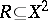defines an an operator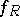on the power set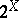, in whichis the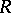-image of the subsetof. This generalizes: each-ary relation ongives rises to an-ary operation on. Thus, any relational structure, comprising a collection of finitary relations on a set, determines a Boolean algebra with operators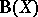based on, known as the complex algebra of(the terminology originates with G. Frobenius in the 1880s, referring to a collection of elements of a group as a "complex" ).

The case of binary relations () is intimately connected with the study of modal logic [a2]. Another connection is with the algebra of topology [a11]: when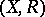is a partial ordering, the operatorobeys the Kuratowski axioms for the closure operator on subsets of a topological space. The general theory of Boolean algebras with operators (BAOs) was introduced by B. Jónsson and A. Tarski [a7], who extended the Stone representation theory that embeds a Boolean algebrainto a certain complete and atomic Boolean algebra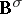, known as the perfect or canonical extension of(cf. also Boolean algebra). They showed that any additive operator onlifts to a completely additive operator onpreserving normality, and also that any normal complete and atomic Boolean algebra with operators is isomorphic to the complex algebra of some relational structure. Consequently, each normal Boolean algebra with operatorsis isomorphically embeddable into the complex algebra of some structure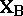.

This representation extends to the level of morphisms: an algebraic homomorphisminduces a certain type of structure-preserving mapping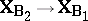, called a bounded morphism. This gives rise to a categorical duality between Boolean algebras with operators and (topological) relational structures that is developed and applied in [a1], [a3]. A survey of the theory of Boolean algebras with operators is given in [a10].

Work in algebraic logic has identified a number of structural properties of varieties (equational classes, cf. Algebraic systems, variety of) of Boolean algebras with operators that are related to natural properties of logical systems. This is surveyed in [a4]. A varietyis:

i) elementary if generated by the complex algebras of some first-order definable class of relational structures;

ii) complex if each of its members is isomorphically embeddable into some complex algebra that belongs to;

iii) canonical if closed under canonical extensions. Every elementary variety is canonical, and every canonical variety is complex. All three notions coincide if the class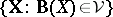of all structures whose complex algebra belongs to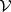is closed under ultrapowers.

The variety generated by the complex algebra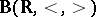(where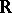denotes the set of real numbers) is complex but not canonical. In general, complexity ofcorresponds to the property of strong completeness of a logical system associated with. The deepest unresolved question in this area is whether every canonical variety must be elementary: ifis closed under canonical extensions, does it follow that there is a first-order definable class of structures whose complex algebras generate? An answer either way would enhance the understanding of the relationship between the equational properties of Boolean algebras with operators and the first-order-definable properties of relational structures.

How to Cite This Entry:
Boolean algebra with operators. Encyclopedia of Mathematics. URL: http://encyclopediaofmath.org/index.php?title=Boolean_algebra_with_operators&oldid=16043
This article was adapted from an original article by R. Goldblatt (originator), which appeared in Encyclopedia of Mathematics - ISBN 1402006098. See original article